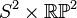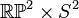# Product of 2-sphere and real projective plane

## Definition

This topological space is defined as the Cartesian product of the 2-sphere$S^2$ and the real projective plane$\R\mathbb{P}^2$ equipped with the product topology. It is denoted$S^2 \times \R\mathbb{P}^2$ or as$\R\mathbb{P}^2 \times S^2$.

## Topological space properties

Property Satisfied? Is the property a homotopy-invariant property of topological spaces? Explanation Corollary properties satisfied/dissatisfied
manifold Yes No product of manifolds is manifold satisfies: metrizable space, second-countable space, and all the separation axioms down from perfectly normal space and monotonically normal space, including normal, completely regular, regular, Hausdorff, etc.
path-connected space Yes Yes path-connectedness is product-closed satisfies: connected space, connected manifold, homogeneous space (via connected manifold, see connected manifold implies homogeneous)
simply connected space No Yes Product of two spaces, one of which (the real projective plane) is not simply connected. dissatisfies: simply connected manifold
rationally acyclic space No Yes The 2-sphere is not rationally acyclic. dissatisfies: acyclic space, weakly contractible space, contractible space
space with Euler characteristic zero No Yes Product of two spaces, neither of which has Euler characteristic zero. Note that Euler characteristic of product is product of Euler characteristics
space with Euler characteristic one No Yes The Euler characteristic is$2 \times 1 = 2$.
compact space Yes No Product of compact spaces, see Tychonoff's theorem satisfies: compact manifold, compact polyhedron, polyhedron (via compact manifold), compact Hausdorff space, and all properties weaker than compactness

## Algebraic topology

### Homology groups

Further information: Kunneth formula, homology of spheres, homology of real projective space

The homology groups with coefficients in integers are as follows:$H_p(S^2 \times \R\mathbb{P}^2; \mathbb{Z}) = \lbrace \begin{array}{rl} \mathbb{Z}, & \qquad p = 0, 2 \\ \mathbb{Z}/2\mathbb{Z}, & \qquad p = 1, 3 \\ 0, & \qquad p > 3 \\\end{array}$